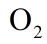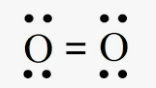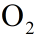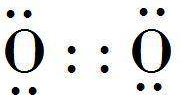o2 lewis structure homework Help at TutorEye

# Best Homework Help For o2 lewis structure

## Top Questions

erature and pressure? Record your answer in scientific notation using 3 significant figures.
View More

##Lewis structure

In Lewis dot structures the electrons are represented as solid dots. In molecule, two oxygen atoms share four electrons and form a double bond completing their respective octets. The four bonded electrons are shown in between two O atoms. The rest of the 4 electrons are present on each oxygen atom as lone pairs.##Lewis structure Sample Questions

Question 1: How many total valence electrons are present in?

a) 4                             b) 8
c) 12                           d) 8

Explanation: Each oxygen atom has 8 electrons, 6 of which are valence electrons. There are two O atoms in, and therefore 12 valence electrons.

Question 2: What kind of bond is present inmolecule?

a) Covalent bond                             b) Ionic bond
c) Co-ordinate bond                         d) None of the above

Explanation: O is a non-metal. Bond formed between two nonmetals is covalent and is formed by sharing of electrons. InBoth O atoms share 4 electrons by forming a double bond. Hence, the bond is covalent

Question 3: What do the two lines represent in Lewis structure of?

a) Three lone pairs                         b) Three electrons
c) Triple bond                                 d) Double bond

Explanation: In the Lewis structure ofThere is a double bond shown as the two parallel lines between the O atoms. This is a triple bond, with each bond formed by a pair of electrons, one from each O atom.

Question 4: Which of the following statements about lewis structure ofis true?

a) There are 8 nonbonding electrons.                         b) There are 2 bonding electrons.
c) There are two lone pairs.                                        d) There are two double bonds.

Answer: Option a) There are 8 nonbonding electrons.

Explanation: Each oxygen atom has 6valence electrons. Out of these 6 , each O atom uses two electrons in bonding with each other and 4 electrons are left on both O atoms. Thus, total of 8 unpaired electrons (four lone pairs) are there in lewis structure of.

Question 5: Oxygen molecule () doesn’t follows the octet rule

a) True                   b) False

Explanation: Each O atom is surrounded by four dots (lone pairs) and two solid lines (double bond), representing another 4 electrons in the double bond. So each O is surrounded by 8 total valence electrons, completing the octet and making it stable.

Question 6: How many bonding electrons are there in?

a) 2                        b) 3
c) 4                        d) 6

Explanation: An oxygen atom has a total of 8 electrons, out of which there are 6 valence electrons. In aMolecule total electrons are 16 and valence electrons are 12. The two electrons from each oxygen atom are shared with each other forming a double bond between two O atoms, thus total bonding electrons in lewis structure ofis 4.

Question 7: How many double bonds are there in lewis structure ofa) 0                        b) 1
c) 2                        d) 3

Explanation: In amolecule two O atoms share 4 electrons with each other forming a double covalent bond between them. Each bond is formed by a pair of electrons and thus 4 electrons give rise to a double bond. In lewis structure the double bond is represented by two solid parallel lines between two O atoms.

Question 8: What do the two dots on an atomic symbol represent in a lewis structure?

a) Lone pair                                           b) Single bond
c) Double bond                                      d) An unpaired electron

Explanation: The lone pair of an atom is represented by two solid dots on the  atomic symbol, in a lewis structure.

Question 9: Valence electrons are-

a) Total electrons in an atom                         b) Electrons in outermost shell
c) Noble gases                                              d) Electrons in outermost subshell

Explanation: Valence electrons are electrons present in the outermost shell of an atom, and can participate in the formation of a chemical bond with other atoms.

Question 10: Pick the incorrect statement aboutlewis structure-

a) There is a double bond present.                                  b) Each oxygen has a lone pair.

c) The nature of bond present is covalent.                       d) Total valence electrons are 12.

Answer: Option b) Each oxygen has a lone pair.

Explanation: Each oxygen atom has 6 valence electrons. Out of these 6 , each O atom uses two electrons in bonding with each other and 4 electrons are left on both O atoms. Thus, total of 8 unpaired electrons (four lone pairs) are there in lewis structure of, with 2 lone pairs each on O atoms.

### What is the correct Lewis structure for o2?

In an oxygen molecule, the two oxygen atoms share a double bond represented by two solid parallel lines between two O atoms. Each bond is formed by a pair of electrons. A double bond means a total of 4 electrons, 2 coming from each oxygen atom. Each oxygen atom is surrounded by 8 electrons and thus has a complete octet and stable state. The four dots on each oxygen atom represent lone pairs of electrons.### What is the Lewis dot structure for?

In Lewis dot structures the electrons are represented as solid dots. Inmolecule, two oxygen atoms share four electrons and form a double bond completing their respective octets. The four bonded electrons are shown in between two O atoms. The rest of the 4 electrons are present on each oxygen atom as lone pairs.### How many bonding electrons are in the lewis structure of?

Each oxygen atom has six valence electrons and they tend to complete their octet of electrons by sharing or receiving electrons from other atoms. In amolecule, the two O atoms share 4 electrons by forming a double bond and complete their octets.So, there are 4 bonding electrons in amolecule.